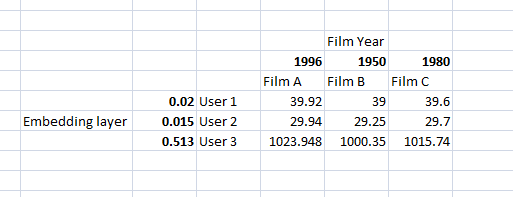# Accessing batch of data from Dataloader in pytorch forward function

I’m trying to improve the collaborating filter presented in the class by adding an embedding layer that will factor in the year of the movie.

The dataloaders (defined as ds) doesn’t include this data, it only stores items,ratings and movies.

Here’s an example in Excel of what I intend to do:
Let me know if you have an idea how this could be achieved.Here’s the code I’m using, the part I don’t understand is highlighted as pseucode:

``````#Loading data
ratings = ratings.merge(movies)

#adding the year of the movie to the DataFrame
ratings['year'] = ratings.title.apply(lambda x: int(x[re.search(r'(\d\d\d\d)', x).start():re.search(r'(\d\d\d\d)', x).start()+4]) if bool(re.search(r'(\d\d\d\d)', x)) else 1990)